0
682

# RRB JE Data Interpretation Questions PDF

Download Top 20 RRB JE Data Interpretation Questions and Answers PDF. RRB JE Maths questions based on asked questions in previous exam papers very important for the Railway JE exam

Instructions

Answer the following using Pie chart and the total chocolates are 350.

Question 1: What is the average number of chocolates received by Ravi,Praful and Srujay ?

a) 80

b) 70

c) 50

d) 60

Question 2: What is the difference between the person receiving highest and lowest number of chocolates ?

a) 105

b) 80

c) 90

d) 70

Instructions

Answer the following using Pie chart and the total cash prize is 21 millions.

Question 3: What is the difference between the averages of cash prize received by Vince,Cena,Kofi combinedly and Orton,Roman,Kofi combinedly ?

a) 0.24 millions

b) 0.36 millions

c) 0.48 millions

d) 0.56 millions

Question 4: What is the average of cash prize won by Vince,Cena and Orton ?

a) 5 millions

b) 4.9 millions

c) 3.8 millions

d) 5.6 millions

Instructions

Answer the following using Pie chart.

Question 5: If the total runs scored by all the players is 500, what is the difference between total runs scored by Kiran,Kohli,Sehwag combinedly and Virat and Rahul combinedly ?

a) 25

b) 50

c) 100

d) 75

Question 6: If the total runs scored by all the players is 500. What is the difference between the runs scored by Kohli and Virat ?

a) 30

b) 50

c) 25

d) 35

Instructions

The number of students appearing for Medical entrance exam from 4 different states over a four year period is shown in the table below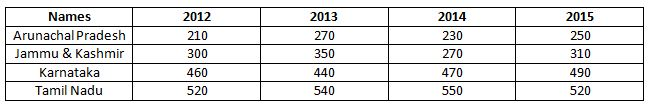Study the table carefully and answer the questions that follow:

Question 7: If we consider each of the numbers for Tamil Nadu as an observation, what is the “mode” of the observations over the four years?

a) 532

b) 530

c) 527

d) 520

Question 8: What the approximate percentage decrease in the number of students appearing from Arunachal Pradesh and Jammu and Kashmir, combined from 2013 to 2014?

a) 5%

b) 10%

c) 15%

d) 20%

Question 9: Which year has the highest number of students appearing for the exam?

a) 2012

b) 2013

c) 2014

d) 2015

Instructions

The marks of 4 students in Maths over four years is as shown in the diagram below.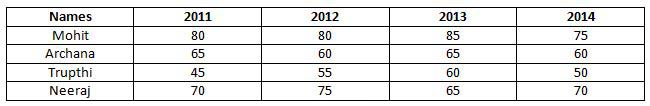Study the table carefully and answer the questions that follow:

Question 10: What is the standard deviation of the scores in 2011?

a) 15.75

b) 12.75

c) 10.75

d) 25.5

Question 11: What is the median score in 2012?

a) 67.5

b) 70

c) 72.5

d) 75

Question 12: Who has the highest range of marks in Maths in these four years?

a) Mohit

b) Archana

c) Trupthi

d) Neeraj

Instructions

The total usage of pesticides and fertilisers in the state of Kerala from 2001 to 2004 is as shown in the diagram below.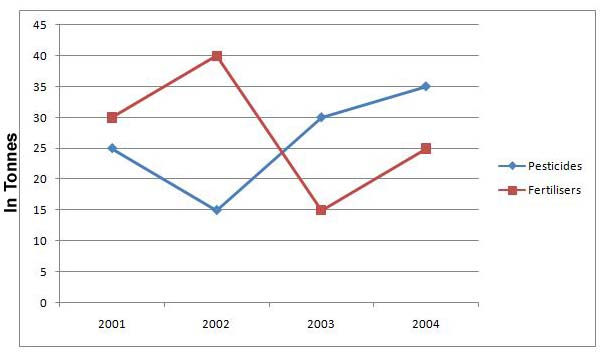Study the chart carefully and answer the questions that follow:
Question 13: If the cost of pesticides is Rs. 2500 per tonne and cost of fertilisers is Rs. 3000 per tonne, on which of the following did the state of Kerala spend more money on, over all the years combined?

a) Fertilizers

b) Pesticides

c) Same on both

d) Can’t be determined

Question 14: What is the percentage decrease in the total amount of pesticides and fertilizers used between 2001 and 2003?

a) 12%

b) 15%

c) 18%

d) 21%

Question 15: In which year is the ratio of fertilisers to pesticides the lowest?

a) 2001

b) 2002

c) 2003

d) 2004

Instructions

The incomes and expenses of four large IT companies for the year 2014 is shown in the diagram below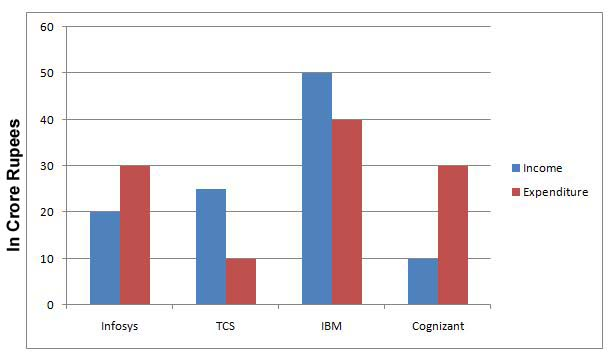Study the chart carefully and answer the questions that follow.

Question 16: By how much is the average income per company less than the average expenditure per company?

a) 125000

b) 1250000

c) 12500000

d) 125000000

Question 17: How many of the four companies have had a surplus in the year 2014?

a) 0

b) 1

c) 2

d) 3

Question 18: Which company has the highest expenditure to income ratio?

a) Infosys

b) TCS

c) IBM

d) Cognizant

Instructions

In a school, the percentage of students who passes the following subjects in different classes is shown in the table below. The number of students appearing from each class is 40 students. Failing in any one subject requires the candidates to get the signature from their parents. Study the table carefully and answer the questions that follow: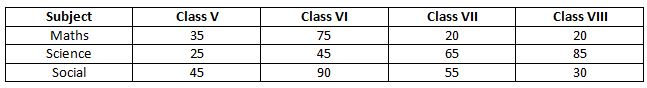Question 19: If we consider the best case scenarios for each class (ie. the lowest possible number of students requiring to get their parents’ signature from each class), which class has the highest number of students who didn’t have to get their parents’ signature?

a) Class V

b) Class VI

c) Class VII

d) Class VIII

Question 20: How many students passed in Maths from all the four classes taken together?

a) 40

b) 50

c) 60

d) 70

Number of chocolates with Ravi=(30/100)*350=105
Number of chocolates with Srujay=(10/100)*350=35
Number of chocolates with Praful=(20/100)*350=70
Average of chocolates receive by these three=(105+35+70)/3
=70

Number of chocolates with Ravi=(30/100)*350=105
Number of chocolates with Srujay=(10/100)*350=35
Difference between them=105-35=70 chocolates

Average of total cash prize received by Vince,Cena,Kofi combinedly=(16+17+29)*21/(300)
Average of total cash prize received by Orton,Roman,Kofi combinedly =(24+14+16)*21/(300)
Difference between the averages=(62-54)*21/(300)
=0.56 millions

Percentage of cash prize won by orton=24%
Percentage of cash prize won by cena=29%
Percentage of cash prize won by vince=17%
Average of these three=(24+29+17)*21/(3*100)
=4.9 millions

Total runs scored by Kiran,Kohli,Sehwag combinedly=(10%+30%+15%)*500=(55/100)*500
=275
Total runs scored by Virat and Rahul=(25%+20%)=(45/100)*500
=225
Difference between them=275-225=50 runs

Percentage of runs scored by Kohli=30%
Percentage of runs scored by Virat=25%
Difference between them is 5% i.e (5/100)*500=25 runs

The values for Tamil Nadu are: 520, 540, 550 and 520.
Since 520 repeats twice (more times than any other value), it is the mode of the observations.

Total students appearing from Arunachal Pradesh and Jammu and Kashmir, combined in 2013 = 270 + 350 = 620

Total students appearing from Arunachal Pradesh and Jammu and Kashmir, combined in 2014 = 230 + 270 = 500
So, percentage decrease = 100* (620 – 500)/620 = 19.35%

The number of students appearing in 2012 = 210 + 300 + 460 + 520 = 1490
The number of students appearing in 2013 = 270 + 350 + 440 + 540 = 1600
The number of students appearing in 2014 = 230 + 270 + 470 + 550 = 1520
The number of students appearing in 2015 = 250 + 310 + 490 + 520 = 1570

So, 2013 has the highest number of students.

The mean of the scores in 2014 = $\frac{80 + 65 + 45 + 70}{4}$ = 65
So, the variance = $\frac{(65 – 80)^2 + (65-65)^2 + (65 – 45)^2 + (65 -70)^2}{4}$ = 650/4 = 162.5
Hence the standard deviation = $\sqrt{162.5}$ = 12.75

The scores for 2012 = 80, 60, 55, 75
Arranging them in the increasing order, we get 55, 60, 75 and 80.
So, the median = $\frac{60 + 75}{2}$ = 67.5

The range of marks (highest – lowest) for Mohit = 85 – 75 = 10
The range of marks (highest – lowest) for Archana= 65 – 60 = 5
The range of marks (highest – lowest) for Trupthi = 60 – 45 = 15
The range of marks (highest – lowest) for Neeraj = 75 – 65 = 10

So, the range is the highest for Trupthi

Total pesticides used = 25 + 15 + 30 + 35 = 105 tons
Total fertilizers used = 30 + 40 + 15 + 25 = 110 tons

Total cost of pesticides = 2500 * 105 = 262,500
Total cost of fertilizers = 3000 * 110 = 330,000

So, the cost of fertilizers is more

Total pesticides and fertilisers used in 2001 = 30 + 25 = 55 tons
Total pesticides and fertilisers used in 2003 = 30 + 15 = 45 tons

So, the percentage change = 100 * (55 – 45) /55 = 18.18%

The ratio of fertilisers to pesticides in 2001 is 30 : 25
The ratio of fertilisers to pesticides in 2002 is 40 : 15
The ratio of fertilisers to pesticides in 2003 is 15 : 30
The ratio of fertilisers to pesticides in 2004 is 25 : 35

So, the lowest ratio is 15:30 (half) in the year 2003.

The average income per company = (20 + 25 + 50 + 10)/4 = 26.25
The average expenditure per company = (30 + 10 + 40 + 30)/4 = 27.50
So, the difference = 27.5 – 26.25 = 1.25 crores

Surplus = Income – Expenditure
The surplus for Infosys = 30 – 20 = 10 crores
The surplus for TCS= 10 – 22 = -12 crores (deficit)
The surplus for IBM = 40 – 50 = – 10 crores (deficit)
The surplus for Cognizant = 30 – 10 = 20 crores
So, 2 companies have surplus and 2 companies have a deficit

The expenditure to income ratio for Infosys is 30 : 20
The expenditure to income ratio for TCS is 10 : 25
The expenditure to income ratio for IBM is 40 : 50
The expenditure to income ratio for Cognizant is 30 : 10

So, Cognizant has the highest expenditure to income ratio.